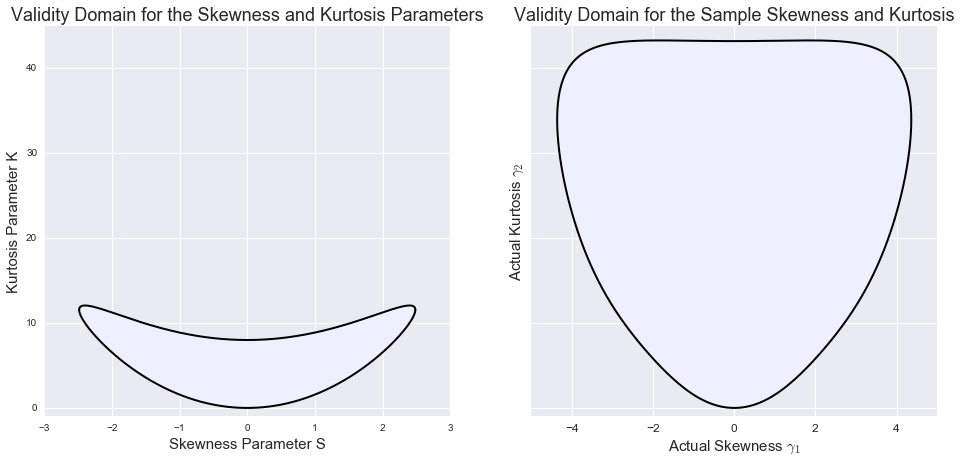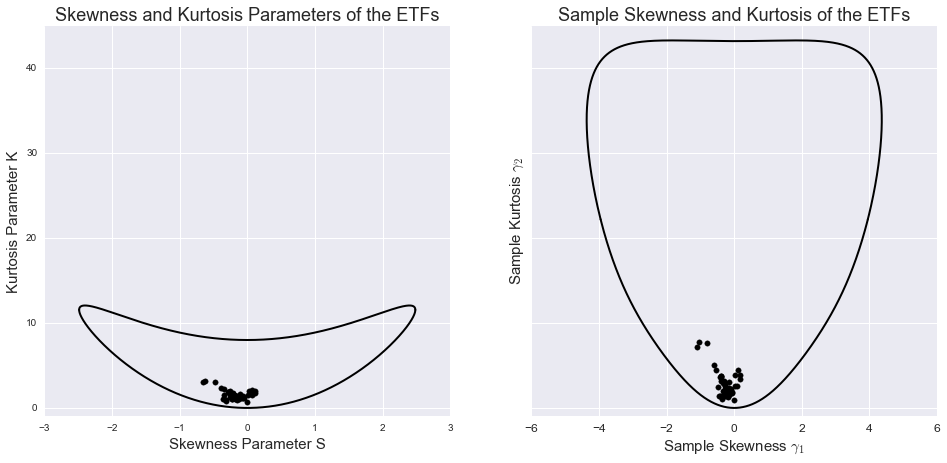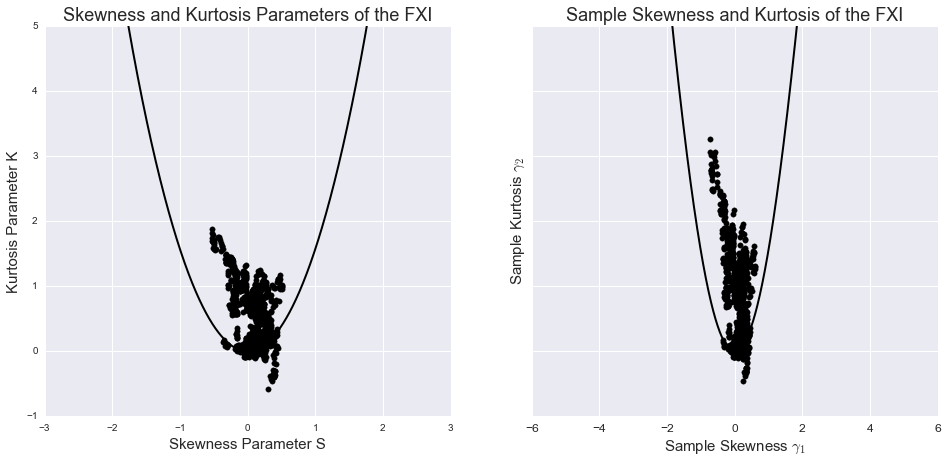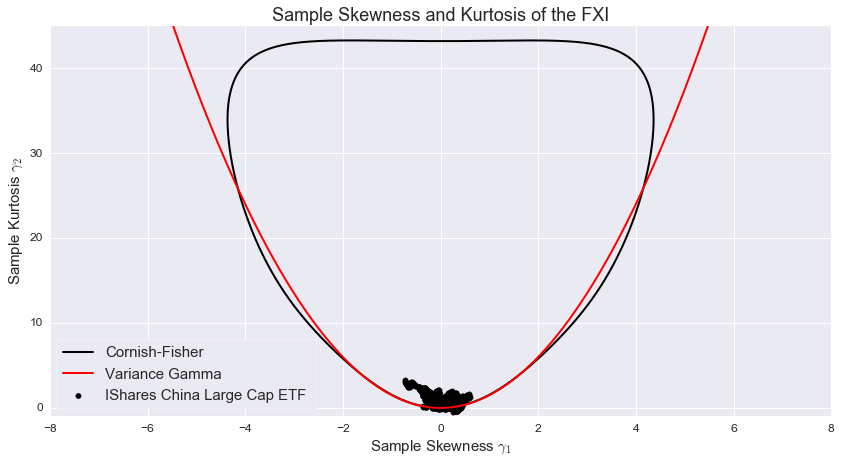### Risk Management Solutions

September 30, 2016

# Anatomy of Cornish-Fisher

### Introduction

The three European Supervisory Authorities (ESMA, EIOPA and EBA) demand that investors are to be informed in a standardised way on the embedded risk of financial products. In their new proposal, these regulators have embraced the concept of the VaR Equivalent Volatility (VEV). The VEV-number is mapped into a market risk-category represented by an integer number in the range $[0 ,7]$. The VaR Equivalent Volatility is derived from a Value at Risk number (VaR). For this VaR measure the Cornish-Fisher expansion is used. In our previous contribution we touched upon this approach. In this whitepaper we examine in detail the validity of the Cornish-Fisher expansion.

### Value at Risk

The Value at Risk is defined for a particular holding period $T$ and confidence level. A 97.5% VaR number for a particular financial instrument or portfolio is the amount such that the probability of a loss exceeding this amount equals 2.5%. For normally distributed log returns, the parametric $97.5\%$-VaR for horizon $T$ is given by the following expression: \begin{equation}\label{eq:VaR} VaR_{0.975} = -\frac{1}{2} \sigma^2T +z^*\sigma \sqrt{T}, \end{equation} with $\sigma$ the volatility of the daily log returns and $T$ the time period for which we want to obtain the VaR. Given the fact that we are dealing with daily returns, $T$ is expressed as a number of business days. The value for $z^*$ is $-1.96$ for a 97.5% confidence level.

### Cornish-Fisher VaR

Since the log returns of financial assets are often skewed and as such not normally distributed, using the VaR formula above will lead to biased results. A possible solution is to use the Cornish-Fisher expansion to estimate quantiles of such a non-normal distribution. This is the proposal on which the risk calculations for packaged retail instruments (PRIIPs) was founded. The Cornish-Fisher expansion, based on four moments, transforms a standard Gaussian variable $z$ into a non Gaussian random variable $Z$, according to the following formula: \begin{equation}\label{eq:CF} Z=z+(z^2-1)\frac{S}{6} + (z^3-3z)\frac{K}{24} - (2z^3-5z)\frac{S^2}{36}, \end{equation} with S a skewness parameter and K an (excess) kurtosis parameter.

It is important to realise that $S$ and $K$ are parameters. As such they can be very different from the actual skew and excess kurtosis of the obtained distribution following the Cornish-Fisher expansion.

To obtain the $2.5\%$-quantile of the transformed distribution, we implement the $2.5\%$ quantile of the standard normal distribution, $z=-1.96$, in the above equation. This gives us
\begin{align*} Z_{0.025}&= -1.96+((-1.96)^2-1)\frac{S}{6} + ((-1.96)^3-3( -1.96))\frac{K}{24} - (2(-1.96)^3-5(-1.96))\frac{S^2}{36}, \\ \text{or}& \\ Z_{0.025}&= -1.96+0.474\ S - 0.0687\ K - 0.146\ S^2. \end{align*}

Using this obtained $Z_{0.025}$ as the $z^*$ quantile into our parametric $97.5\%$-VaR formula, we obtain the Cornish-Fisher formula for the VaR

\begin{equation} VaR_{0.975} = -\frac{1}{2} \sigma^2T + (-1.96+0.474\ S - 0.0687\ K - 0.146\ S^2)\sigma \sqrt{T}. \end{equation}

This is the formula for the VaR that is imposed by the European Supervisory Authorities in the PRIIPs regulations (March 2016)

### Skewness and Kurtosis Parameters

Using the Cornish-Fisher expansion we transform a standard normal distribution into a non-normal distribution. The skewness and kurtosis of this transformed distribution are called the actual (sample) skewness and the actual kurtosis. The actual skewness and actual kurtosis do not correspond with the skewness and kurtosis parameters in the Cornish-Fisher expansion. This raises the question what values to use for the skewness parameter $S$ and kurtosis parameter $K$.

Denoting the actual skewness with $\gamma_1$ and the actual excess kurtosis with $\gamma_2$, we have the following relations between the actual and parameter skewness and kurtosis:

\begin{align*} \gamma_1 & = \dfrac{S-\frac{76}{216}S^3 + \frac{85}{1296}S^5 + \frac{1}{4}KS - \frac{13}{144}KS^3 + \frac{1}{32}K^2S}{\left(1 + \frac{1}{96}K^2 + \frac{25}{1296}S^4 - \frac{1}{36}KS^2\right)^{1.5}} \\ \\ \gamma_2 & =\left[\dfrac{3 + K + \frac{7}{16}K^2 + \frac{3}{32}K^3 + \frac{31}{3072}K^4 - \frac{7}{216}S^4 - \frac{25}{486}S^6 + \frac{21665}{559872} S^8 - \frac{7}{12}KS^2 + \frac{113}{452}KS^4 - \frac{5155}{46656}KS^6 - \frac{7}{24}K^2S^2 + \frac{2455}{20736}K^2S^4 - \frac{65}{1152}K^3S^2}{\left(1 + \frac{1}{96}K^2 + \frac{25}{1296}S^4 - \frac{1}{36}KS^2\right)^2} \right]-3 \end{align*}

We would like to have the actual skewness $\gamma_1$ and the actual kurtosis $\gamma_2$ corresponding with the sample skewness and the sample kurtosis of the historical log returns. The above equations can be solved numerically to obtain the skewness parameter $S$ and the kurtosis parameter $K$ using the sample skewness and kurtosis for $\gamma_1$ and $\gamma_2$. For small values of the skewness and excess kurtosis, the actual and parameter skewness and kurtosis will coincide. The table below illustrates the values of actual skewness and kurtosis ($\gamma_1, \gamma_2$ ) with corresponding skewness and kurtosis parameters $(S,K)$. A problem with these equations is that they do not have a single solution. The values found for the skewness and kurtosis parameters depend on the starting values used in the numerical optimization.

ACTUAL PARAMETER
$\gamma_1$ $\gamma_2$ $S$ $K$
0 0 0 0
0.1 0.2 0.0958 0.1872
-0.2 0.5 -0.1821 0.4317
1 2 0.8833 3.5875
-1.5 8 -0.9320 3.5875

### Domain of validity

Although the Cornish-Fisher expansion gives a good method to transform a normal distribution into a non-normal distribution, there are limits on the validity of this expansion. The transformation must be increasing such that the order of the quantiles of the distributions is conserved. This is the case if the skewness parameter $S$ is in absolute values smaller than $\sqrt{2}-1 \approx 2.4853$ and the kurtosis parameter $K$ has values between $\dfrac{36 + 11 S^2 - \sqrt{324-54S^2+\frac{1}{4}S^4}}{9}$ and $\dfrac{36 + 11 S^2 + \sqrt{324-54S^2+\frac{1}{4}S^4}}{9}.$ For a skewness parameter $S$ equal to zero, this corresponds with a kurtosis parameter $K$ between $0$ and $8$. The maximum possible kurtosis parameter $K$ is $11.55$.

The limits on the skewness and kurtosis parameters also imply limits on the actual skewness $\gamma_1$ and the actual excess kurtosis $\gamma_2$. Both domains of validity are visualised in the figure below. The actual skewness $\gamma_1$ is limited by $-4.36$ and $4.36$ while the actual excess kurtosis can take values in the range $[0, 43.28]$.This is where it gets interesting since we can use the domains above to verify if financial instruments easily verify these conditions.

### Example1

An analysis was done on a sample of $62$ exchange traded funds (ETF) with a US listing (Calculation Date : Sep 30, 2016). The required minimum market cap of the ETFs was \$10 bn. A total of 13 of the selected ETFs were bond-based while the remaining$49$instruments were equity ETFs. We investigated the skewness$\gamma_1$and excess kurtosis$\gamma_2$of the log returns to see if the domain of validity is large enough to use the Cornish-Fisher expansion in practical VaR calculations. For all ETFs in our sample the actual skewness and kurtosis were calculated using a 5 year history of daily log returns. The results illustrate that all the ETFs are inside the validity domain. The corresponding skewness and kurtosis parameters ($S$and$K$) are logicaly also inside their validity domain. So for all these ETFs the Cornish-Fisher expansion can be used.### Example 2 The verification on all the ETFs in our previous sample was based on skew and kurtosis values based on 5 year daily returns. Ths outcome of the analysis changes when considering a different observation period. As an example, we retained the IShares China Large Cap ETF (FXI) and studied the validity to use Cornish Fisher to determine VaR using a rolling 90-Day window.The Figure above now points out, that for some time periods, the oberved values for the sample skew and kurtosis fell outside the validity domain. In this particular case, the VaR result and resulting VEV-number would have been flawed. #### Matching up with Variance Gamma In subsequent research we will investigate if other stochastic models could be a validate candidate to stand up against a VaR based on Cornish-Fisher. A possible choice is the Variance Gamma (VG) model. There is no diffusion component in a VG process and it is therefore a pure jump process. The jumps take place at random times. In the VG setting there is also a validity domain linking excess kurtosis and skew. The validity domain for the excess kurtosis ($\gamma_2$) for a particular skew ($\gamma_1\$) is given now by the following equation : $\gamma_2 > \frac{1}{2}(6 + 3\gamma_1^2)-3$
The Figure below compares the wider domain of Variance Gamma with the validity domain of Cornish Fisher. Moreover, the VG process comes with the elegant property that its parameters can be calibrated from listed option prices.Reuters

#### References

Maillard, Didier. A User’s Guide to the Cornish Fisher Expansion. 2012. Electronic copy available at http://ssrn.com/abstract=1997178

Guillaume, Florence and Schoutens, Wim . A Moment Matching Market Implied Calibration. Electronic copy available at http://ssrn.com/abstract=2021466

EIOPA. Final Draft Regulatory Technical Standards. 31 March 2016.